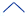﻿ Group functions

# Group functions

In SQL, a small set of group functions have special status. This help topic outlines only the principal characteristics of group functions. For details on syntax, semantics and nesting, consult any online SQL tutorial.

By contrast with inline functions, which operate on rows and column values one-by-one, and return a modified value for each row, group functions operate on the entire set of result rows and then yield a single result value. In the following query, the WHERE clause perhaps causes a set of 100 rows to be passed to MAX(), and then the outcome of applying MAX( ) reduces the number of result values from 100 to a single value:

 SELECT    MAX( amount ) FROM      order WHERE     month = 'Feb 2020'

The result of this query could be:

 499303900

SQL has 7 group functions:

 Group function Returns Empty-set result value * AVG(  ) The average of the row values passed. The NULL value COUNT(  ) The number of rows passed (independently of values in those rows). 0 (zero) MAX(  ) The greatest of the values passed. The NULL value MIN(  ) The least of the values passed. The NULL value STDDEV(  ) The standard deviation for the set of values passed. The NULL value SUM(  ) The sum of the values passed. The NULL value VARIANCE(  ) The statistical variance for the set of values passed. The NULL value

* The empty-set result value is the value that is the result of applying the group function to an empty row set.Group functions and GROUP BY, HAVINGNULL input valuesNULL output values

See also

Column expressions

Function calls

SQL functions